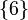# A5 in A6

This article is about a particular subgroup in a group, up to equivalence of subgroups (i.e., an isomorphism of groups that induces the corresponding isomorphism of subgroups). The subgroup is (up to isomorphism) alternating group:A5 and the group is (up to isomorphism) alternating group:A6 (see subgroup structure of alternating group:A6).
VIEW: Group-subgroup pairs with the same subgroup part | Group-subgroup pairs with the same group part | All pages on particular subgroups in groups

This article describes a subgroup$H$ in a group$G$, where$G$ is alternating group:A6, the alternating group on the set$\{ 1,2,3,4,5,6\}$, and$H = H_6$ is the subgroup of$G$ comprising those permutations that fix$\{ 6 \}$.$H$ can be identified with alternating group:A5 via its action on$\{ 1,2,3,4,5\}$.$H$ has five other conjugate subgroups:

•$H_1$ is the subgroup of$G$ fixing$\{ 1 \}$
•$H_2$ is the subgroup of$G$ fixing$\{ 2 \}$
•$H_3$ is the subgroup of$G$ fixing$\{ 3 \}$
•$H_4$ is the subgroup of$G$ fixing$\{ 4 \}$
•$H_5$ is the subgroup of$G$ fixing$\{ 5 \}$

## Arithmetic functions

Function Value Explanation Comment
order of the group 360
order of the subgroup 60
index of the subgroup 6
size of conjugacy class 6
number of conjugacy classes in automorphism class 2

## GAP implementation

### Construction of group-subgroup pair

The group-subgroup pair can be constructed using the AlternatingGroup function:

G := AlternatingGroup(6); H := AlternatingGroup(5);